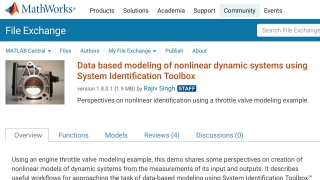# Data based modeling of nonlinear dynamic systems using System Identification ToolboxIntermediate
Software
Demonstration

Using an engine throttle valve modeling example, this demo shares some perspectives on creation of nonlinear models of dynamic systems from the measurements of its input and outputs. It describes useful workflows for approaching the task of data-based modeling using System Identification Toolbox™ . Two modeling approaches are described:
1. Black box modeling: case where you cannot derive the exact mathematical representation of the system from physical considerations; the form of the model as well as the values of its coefficients is extracted from data.
2. Grey-box modeling: the equations of motion relating the input and output variables are known, but the values of various physical constants in the equations are unknown; the data is then used to find the values of those unknowns only.

The emphasis is on the black box modeling approach. It is shown that even though no a priori knowledge of model structure is required, it is often helpful to have some intuition about the nature of the system and to use this knowledge to fine-tune the configuration of model structures.

This resource is included in the following topics and journeys: